# The MI Procedure

### Input Data Sets

Subsections:

You can specify the input data set with missing values by using the DATA= option in the PROC MI statement. When an MCMC method is used, you can specify the data set that contains the reference distribution information for imputation with the INEST= option, the data set that contains initial parameter estimates for the MCMC method with the INITIAL=INPUT= option, and the data set that contains information for the prior distribution with the PRIOR=INPUT= option in the MCMC statement.

When the ADJUST option is specified in the MNAR statement, you can use the PARMS= option to specify the data set that contains adjustment parameters for the sensitivity analysis.

#### DATA=SAS-data-set

The input DATA= data set is an ordinary SAS data set that contains multivariate data with missing values.

#### INEST=SAS-data-set

The input INEST= data set is a TYPE=EST data set and contains a variable _Imputation_ to identify the imputation number. For each imputation, PROC MI reads the point estimate from the observations with _TYPE_=‘PARM’ or _TYPE_=‘PARMS’ and the associated covariances from the observations with _TYPE_=‘COV’ or _TYPE_=‘COVB’. These estimates are used as the reference distribution to impute values for observations in the DATA= data set. When the input INEST= data set also contains observations with _TYPE_=‘SEED’, PROC MI reads the seed information for the random number generator from these observations. Otherwise, the SEED= option provides the seed information.

#### INITIAL=INPUT=SAS-data-set

The input INITIAL=INPUT= data set is a TYPE=COV or CORR data set and provides initial parameter estimates for the MCMC method. The covariances derived from the TYPE=COV/CORR data set are divided by the number of observations to get the correct covariance matrix for the point estimate (sample mean).

If TYPE=COV, PROC MI reads the number of observations from the observations with _TYPE_=‘N’, the point estimate from the observations with _TYPE_=‘MEAN’, and the covariances from the observations with _TYPE_=‘COV’.

If TYPE=CORR, PROC MI reads the number of observations from the observations with _TYPE_=‘N’, the point estimate from the observations with _TYPE_=‘MEAN’, the correlations from the observations with _TYPE_=‘CORR’, and the standard deviations from the observations with _TYPE_=‘STD’.

#### PARMS= SAS-data-set

The input PARMS= data set is an ordinary SAS data set that contains adjustment parameters for imputed values of the specified imputed variables.

The PARMS= data set contains variables _Imputation_ for the imputation number, the SHIFT= or DELTA= variable for the shift parameter, and the SCALE= variable for the scale parameter. Either the shift or scale variable must be included in the data set.

#### PRIOR=INPUT=SAS-data-set

The input PRIOR=INPUT= data set is a TYPE=COV data set that provides information for the prior distribution. You can use the data set to specify a prior distribution for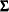of the form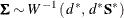where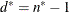is the degrees of freedom. PROC MI reads the matrix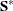from observations with _TYPE_=‘COV’ and reads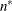from observations with _TYPE_=‘N’.

You can also use this data set to specify a prior distribution for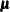of the form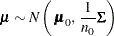PROC MI reads the mean vector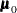from observations with _TYPE_=‘MEAN’ and reads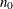from observations with _TYPE_=‘N_MEAN’. When there are no observations with _TYPE_=‘N_MEAN’, PROC MI readsfrom observations with _TYPE_=‘N’.